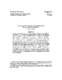## On an Inexpensive Triangular Approximation to the Singular Value Decomposition##### Files
CS-TR-3840.ps(215.72 KB)
In this paper we introduce a new decomposition called the pivoted QLP~decomposition. It is computed by applying pivoted orthogonal triangularization to the columns of the matrix $X$ in question to get an upper triangular factor $R$ and then applying the same procedure to the rows of $R$ to get a lower triangular matrix $L$. The diagonal elements of $R$ are called the R-values of $X$; those of $L$ are called the L-values. Numerical examples show that the L-values track the singular values of $X$ with considerable fidelity\,---\,far better than the R-values. At a gap in the L-values the decomposition provides orthonormal bases of analogues of row, column, and null spaces provided of $X$. The decomposition requires no more than twice the work required for a pivoted QR~decomposition. The computation of $R$ and $L$ can be interleaved, so that the computation can be the rows of $R$ to get a lower triangular matrix $L$. The diagonal elements of $R$ are called the R-values of $X$; those of $L$ are called the L-values. Numerical examples show that the L-values track the singular values of $X$ with considerable fidelity\,---\,far better than the R-values. At a gap in the L-values the decomposition provides orthonormal bases of analogues of row, column, and null spaces provided of $X$. The decomposition requires no more than twice the work required for a pivoted QR~decomposition. The computation of $R$ and $L$ can be interleaved, so that the computation can be terminated at any suitable point, which makes the decomposition especially suitable for low-rank determination problems. The interleaved algorithm also suggests a new, efficient 2-norm estimator. (Also cross-referenced as UMIACS-TR-97-75)